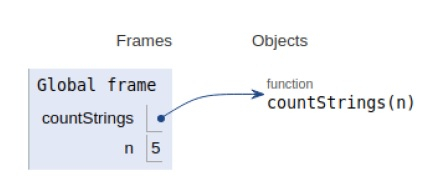# Python Program to Count number of binary strings without consecutive 1’

Problem statement − We are given a positive integer N, we need to count all possible distinct binary strings available with length N such that no consecutive 1’s exist in the string.

Now let’s observe the solution in the implementation below −

## Example

Live Demo

# count the number of strings
def countStrings(n):
a=[0 for i in range(n)]
b=[0 for i in range(n)]
a = b = 1
for i in range(1,n):
a[i] = a[i-1] + b[i-1]
b[i] = a[i-1]
return a[n-1] + b[n-1]
# main
n=5
print("The number of strings: ",countStrings(n))

## Output

The number of strings: 13All the variables are declared in the local scope and their references are seen in the figure above.

## Conclusion

In this article, we have learned about how we can make a Python Program to Count number of binary strings without consecutive 1’